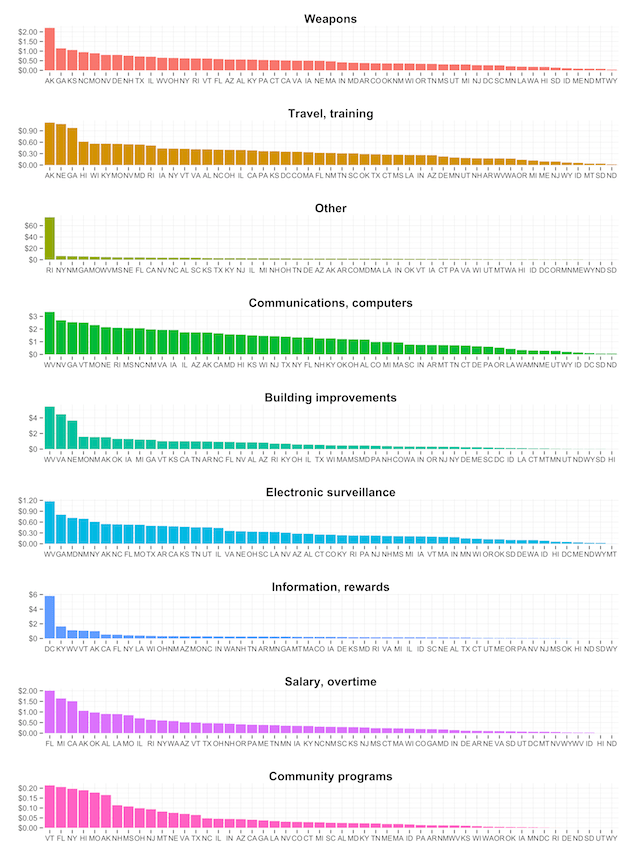Want to share your content on R-bloggers? click here if you have a blog, or here if you don't.

The Washingon Post did another great story+vis, this time on states Spending seized assets.

Since 2008, about 5,400 police agencies have spent \$2.5 billion in proceeds from cash and property seized under federal civil forfeiture laws. Police suspected the assets were linked to crime, although in 81 percent of cases no one was indicted.

Their interactive visualization lets you drill down into each state to examine the spending in each category. Since the WaPo team made the data available [JSON] I thought it might be interesting to take a look at a comparison across states (i.e. who are the “big spenders” of this siezed hoarde). Here’s a snippet of the JSON:

```{"states": [ { "st": "AK", "stn": "Alaska", "total": 8470032, "cats": [{ "weapons": 1649832, "electronicSurv": 402490, "infoRewards": 760730, "travTrain": 848128, "commPrograms": 121664, "salaryOvertime": 776766, "other": 1487613, "commComp": 1288439, "buildImprov": 1134370 }], "agencies": [ { "aid": "AK0012700", "aname": "Airport Police & Fire Ted Stevens Anch Int'L Arpt", "total": 611553, "cats": [{ "weapons": 214296, "travTrain": 44467, "other": 215464, "commComp": 127308, "buildImprov": 10019 }] }, { "aid": "AK0010100", "aname": "Anchorage Police Department", "total": 3961497, "cats": [{ "weapons": 1104777, "electronicSurv": 94741, "infoRewards": 743230, "travTrain": 409474, "salaryOvertime": 770709, "other": 395317, "commComp": 249220, "buildImprov": 194029 }] },```

Getting the data was easy (in R, of course!). Let’s setup the packages we’ll need:

```library(data.table) library(dplyr) library(tidyr) library(ggplot2) library(scales) library(grid) library(statebins) library(gridExtra)```

We also need `jsonlite`, but only to parse the data (which I’ve downloaded locally), so we’ll just do that in one standalone line:

`data <- jsonlite::fromJSON("all.json", simplifyVector=FALSE)`

It’s not fair (or valid) to just compare totals since some states have a larger population than others, so we’ll show the data twice, once in raw totals and once with a per-capita lens. For that, we’ll need population data:

```pop <- read.csv("http://www.census.gov/popest/data/state/asrh/2013/files/SCPRC-EST2013-18+POP-RES.csv", stringsAsFactors=FALSE) colnames(pop) <- c("sumlev", "region", "divison", "state", "stn", "pop2013", "pop18p2013", "pcntest18p") pop\$stn <- gsub(" of ", " Of ", pop\$stn)```

We have to fix the `District of Columbia` since the WaPo data capitalizes the `Of`.

Now we need to extract the agency data. This is really straightforward with some help from the `data.table` package:

```agencies <- rbindlist(lapply(data\$states, function(x) { rbindlist(lapply(x\$agencies, function(y) { data.table(st=x\$st, stn=x\$stn, aid=y\$aid, aname=y\$aname, rbindlist(y\$cats)) }), fill=TRUE) }), fill=TRUE)```

The `rbindlist` `fill` option is super-handy in the event we have varying columns (and, we do in this case). It’s also wicked-fast.

Now, we use some `dplyr` and `tidyr` to integrate the population information and summarize our data (OK, we cheat and use `melt`, but some habits are hard to break):

```c_st <- agencies %>% merge(pop[,5:6], all.x=TRUE, by="stn") %>% gather(category, value, -st, -stn, -pop2013, -aid, -aname) %>% group_by(st, category, pop2013) %>% summarise(total=sum(value, na.rm=TRUE), per_capita=sum(value, na.rm=TRUE)/pop2013) %>% select(st, category, total, per_capita)```

Let’s use a series of bar charts to compare state-against state. We’ll do the initial view with just raw totals. There are 9 charts, so this graphic scrolls a bit and you can select it to make it larger:

```# hack to ordering the bars by kohske : http://stackoverflow.com/a/5414445/1457051 #####   c_st <- transform(c_st, category2=factor(paste(st, category))) c_st <- transform(c_st, category2=reorder(category2, rank(-total)))   # pretty names #####   levels(c_st\$category) <- c("Weapons", "Travel, training", "Other", "Communications, computers", "Building improvements", "Electronic surveillance", "Information, rewards", "Salary, overtime", "Community programs") gg <- ggplot(c_st, aes(x=category2, y=total)) gg <- gg + geom_bar(stat="identity", aes(fill=category)) gg <- gg + scale_y_continuous(labels=dollar) gg <- gg + scale_x_discrete(labels=c_st\$st, breaks=c_st\$category2) gg <- gg + facet_wrap(~category, scales = "free", ncol=1) gg <- gg + labs(x="", y="") gg <- gg + theme_bw() gg <- gg + theme(strip.background=element_blank()) gg <- gg + theme(strip.text=element_text(size=15, face="bold")) gg <- gg + theme(panel.margin=unit(2, "lines")) gg <- gg + theme(panel.border=element_blank()) gg <- gg + theme(legend.position="none") gg```

## Comparison of Spending Category by State (raw totals)There are definitely a few, repeating “big spenders” in that view, but is that the real story? Let’s take another look, but factoring in state population:

```# change bar order to match per-capita calcuation #####   c_st <- transform(c_st, category2=reorder(category2, rank(-per_capita)))   # per-capita bar plot #####   gg <- ggplot(c_st, aes(x=category2, y=per_capita)) gg <- gg + geom_bar(stat="identity", aes(fill=category)) gg <- gg + scale_y_continuous(labels=dollar) gg <- gg + scale_x_discrete(labels=c_st\$st, breaks=c_st\$category2) gg <- gg + facet_wrap(~category, scales = "free", ncol=1) gg <- gg + labs(x="", y="") gg <- gg + theme_bw() gg <- gg + theme(strip.background=element_blank()) gg <- gg + theme(strip.text=element_text(size=15, face="bold")) gg <- gg + theme(panel.margin=unit(2, "lines")) gg <- gg + theme(panel.border=element_blank()) gg <- gg + theme(legend.position="none") gg```

## Comparison of Spending Category by State (per-capita)That certainly changes things! Alaska, West Virginia, and D.C. definitely stand out for “Weapons”, “Other” & “Information”, respectively, (what’s Rhode Island hiding in “Other”?!) and the “top 10″ in each category are very different from the raw total’s view. We can look at this per-capita view with the `statebins` package as well:

```st_pl <- vector("list", 1+length(unique(c_st\$category)))   j <- 0 for (i in unique(c_st\$category)) { j <- j + 1 st_pl[[j]] <- statebins_continuous(c_st[category==i,], state_col="st", value_col="per_capita") + scale_fill_gradientn(labels=dollar, colours=brewer.pal(6, "PuBu"), name=i) + theme(legend.key.width=unit(2, "cm")) } st_pl[[1+length(unique(c_st\$category))]] <- list(ncol=1)   grid.arrange(st_pl[], st_pl[], st_pl[], st_pl[], st_pl[], st_pl[], st_pl[], st_pl[], st_pl[], ncol=3)```

## Per-capita “Statebins” view of WaPo Seizure Data

(Doing this exercise also showed me I need to add some flexibility to the `statebins` package).

The gist shows how to build a top-level category data table (along with the rest of the code in this post). I may spin this data up into an interactive D3 visualization in the next week or two (as I think it might work better than large faceted bar charts), so stay tuned!

A huge thank you to the WaPo team for making data available to others. Go forth and poke at it with your own questions and see what you can come up with (perhaps comparing by area of state)!